# Algebra II : Graphing Hyperbolic Functions

## Example Questions

### Example Question #1 : Graphing Hyperbolic Functions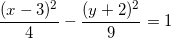In which direction does the graph of the above hyperbola open?

Possible Answers:

vertical

horizontal

Correct answer:

horizontal

Explanation:

To determine which direction a hyperbola opens, first get the equation into standard form for a conic section: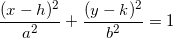This equation gives us a hyperbola when the coefficient in front of either the x-squared or the y-squared term (but not both!) is negative. In this problem, the coefficient in front of the x-squared term is positive, but the coefficient of the y-squared term is negative. Here are the rules for hyperbola directions:

• If the coefficient of the x-squared term is positive but the coefficient of the y-squared term is negative, this is a hyperbola that opens horizontally.
• If the coefficient of the x-squared term is negative but the coefficient of the y-squared term is positive, this is a hyperbola that opens vertically.

Thus, we have a graph of a horizontal hyperbola.

### Example Question #2 : Graphing Hyperbolic Functions

What are the vertices of this hyperbola?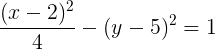Possible Answers: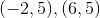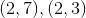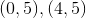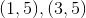Correct answer:Explanation:

The first thing we need to find for this hyperbola is the center. This is simply the point where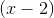and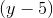both equal, which is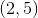. Since theterm is the positive one, the hyperbola opens horizontally, which means we need to look at the denominator of thatterm.

The denominator iswhich is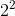, so our vertices are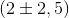, or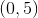and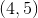.

### Example Question #3 : Graphing Hyperbolic Functions

Write the expression for this hyperbola in standard form: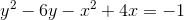Possible Answers: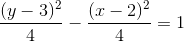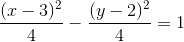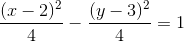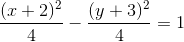Correct answer:Explanation:

The standard form of a hyperbola is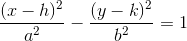or the similar form with a positiveterm and negativeterm. So to start out getting this equation in standard form, we must complete the square on the quadratics inand.

the coefficient ofis, so completing the square we get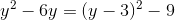and similarly withwe get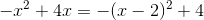and so our starting expression can be written as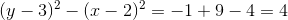Dividing byon both sides we get the standard representation of the hyperbola,### Example Question #4 : Graphing Hyperbolic Functions

Find the vertices of the following hyperbolic function: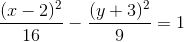Possible Answers: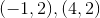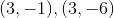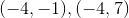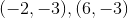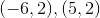Correct answer:Explanation:

We start by noticing that our hyperbola is given in the following form:In order to determine the vertices of the hyperbola, we must first locate its center. Using the standard form given above, we know the center of the hyperbola occurs at the point (h,k), so for the equation given in the problem the center is at (2,-3). Now that we know the location of the hyperbola's center, our next step is to determine how far the vertices are from the center of the hyperbola. Looking at our equation, we can see it is in the form where the x term occurs first, which means the hyperbola opens left and right as opposed to up and down (which would be the case if the y term occurred first. Given this information, we know the vertices of the hyperbola are going to be a distanceto the left and right of the center. The denominator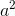of the x term in the hyperbolic equation is 16, which meansis equal to 4, so the vertices of the hyperbola will be 4 units to the left and right of the center (2,-3), which gives us: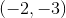and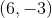### All Algebra II Resources